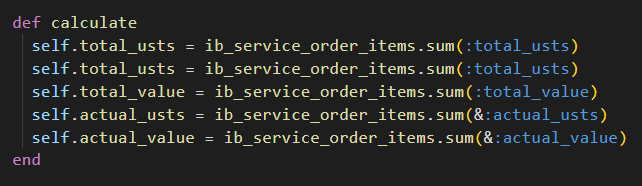# What is the difference between model.sum(&:column) and model.sum(:column)?

Hi! Newbie question so pleae bear with me.

What is the difference between model.sum(&:column) and model.sum(:column)?

I ask because I saw this codeIn a redmine (rails) plugin.

First one is a Rails method that will be converted into a SQL query and return the sum. Second one is a Ruby method that cause Rails to load every order_item in memory, pass them to Ruby to do the sum.

Take a look at the query log and the difference will be obvious:

``````> Order.where(paid_at: Date.current..).sum(:item_total)
# DEBUG -- : Order Sum   SELECT SUM("orders"."item_total") FROM "orders" WHERE "orders"."paid_at" >= \$1  [["paid_at", "2023-07-12"]]

> Order.where(paid_at: Date.current..).sum(&:item_total)
# DEBUG -- : Order Load  SELECT "orders".* FROM "orders" WHERE "orders"."paid_at" >= \$1  [["paid_at", "2023-07-12"]]
``````

TL:DR: If you have not accessed `ib_service_order_items` before in your code, `(:total_usts)` will be faster (no need to load every item in memory before summing). If you have accessed `ib_service_order_items` before in your code, `(&:total_usts)` will be faster (not need for the extra query, just use the objects in memory.

3 Likes

`model.sum(&:column)` is used when you want to call a method (`column`) on each element of `model` and sum up the results. `model.sum(:column)` is used when you want to calculate the sum of a specific column (`:column`) in `model`.

That was quite clear! Thanks!

1 Like

This syntax calculates the sum of the specified column directly within the database using SQL. It generates a SQL query that performs the sum operation on the specified column in the associated table and returns the result. Example:

total_amount = Order.sum(:total_amount)

**`model.sum(&:column)`: This syntax uses Ruby’s `Enumerable#sum` method in conjunction with a symbol to sum up a specific attribute (column) for a collection of records in memory. It fetches all the records from the database for the model and then iterates over them in memory to calculate the sum of the specified attribute.